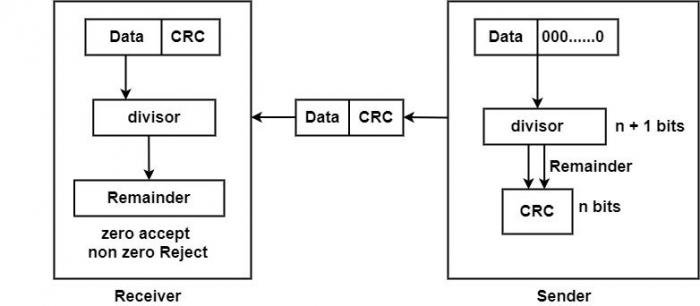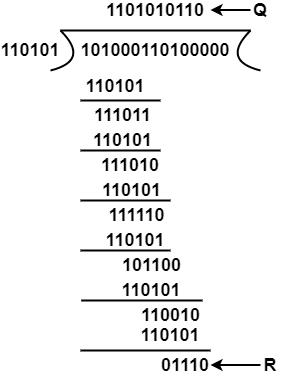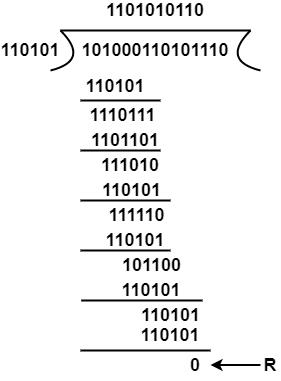# Explain the Cyclic Redundancy Checks (CRCs)

The Cyclic Redundancy Checks (CRC) is the most powerful method for Error-Detection and Correction. It is given as a kbit message and the transmitter creates an (n – k) bit sequence called frame check sequence. The out coming frame, including n bits, is precisely divisible by some fixed number. Modulo 2 Arithmetic is used in this binary addition with no carries, just like the XOR operation.

Redundancy means duplicacy. The redundancy bits used by CRC are changed by splitting the data unit by a fixed divisor. The remainder is CRC.

Qualities of CRC

• It should have accurately one less bit than the divisor.

• Joining it to the end of the data unit should create the resulting bit sequence precisely divisible by the divisor.

CRC generator and checker## Process

• A string of n 0s is added to the data unit. The number n is one smaller than the number of bits in the fixed divisor.

• The new data unit is divided by a divisor utilizing a procedure known as binary division; the remainder appearing from the division is CRC.

• The CRC of n bits interpreted in phase 2 restores the added 0s at the end of the data unit.

Example

Message D = 1010001101 (10 bits)

Predetermined P = 110101 (6 bits)

FCS R = to be calculated 5 bits

Hence, n = 15 K = 10 and (n – k) = 5

The message is generated through 25:accommodating 1010001101000

The product is divided by P.The remainder is inserted to 25D to provide T = 101000110101110 that is sent.

Suppose that there are no errors, and the receiver gets T perfect. The received frame is divided by P.Because of no remainder, there are no errors.